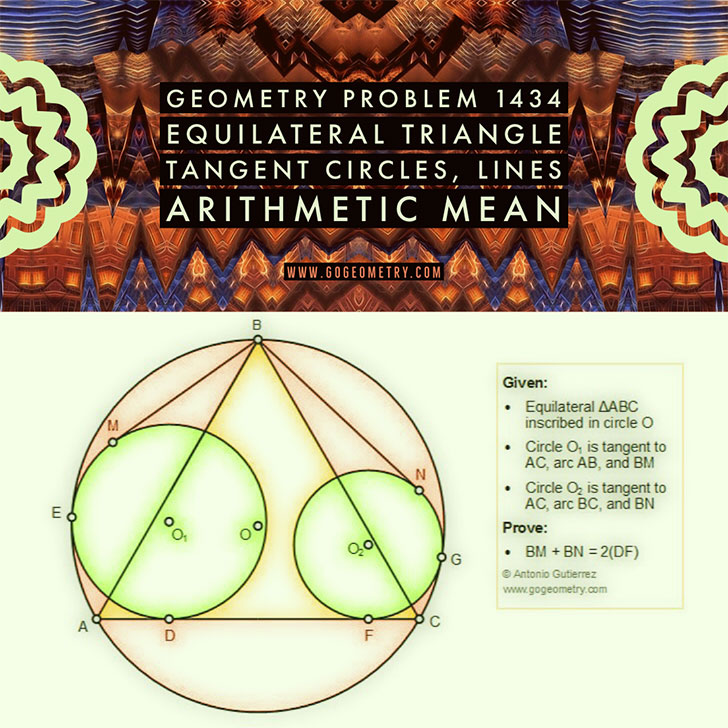# Geometry Problem 1434# Geometry Problem 1434: Equilateral Triangle, Tangent Circles, Tangent Lines, Arithmetic Mean, Measurement

### Proposition

The figure shows an equilateral triangle ABC inscribed in a circle O. Circle O1 is tangent to AC at D, arc AB at E, and BM at M. Circle O2 is tangent to AC at F, arc BC at G, and BN at N. Prove that DF is the arithmetic mean of BM and BN: BM + BN = 2(DF)### Geometric Art using iPad or Mobile Apps

Geometric art is a form of art based on the use and application of geometric figures. A geometric figure is any set or combination of points, lines, surfaces and solids.

A mobile app or mobile application software is a computer program designed to run on smartphones and tablet computers.